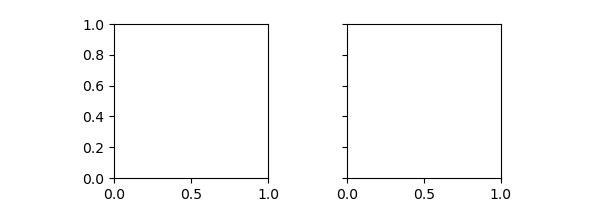``````fig, axes = plt.subplots(1, 2, figsize=(6, 2), squeeze=False, sharex=True, sharey=True)
for i in range(1):
for j in range(2):
axes[i, j].set(aspect="equal")
plt.show()
``````I want to have 2 1x1 square plot stacked horizonally together, but `aspect="equal"` and `subplots_adjust(wspace=0)` are conflict, so that I have to change the `figsize` to make them stack together.

for example, this works:

``````fig, axes = plt.subplots(1, 2, figsize=(3.9, 2), squeeze=False, sharex=True, sharey=True)
for i in range(1):
for j in range(2):
axes[i, j].set(aspect="equal")
If you didn’t want to use a layout engine for some reason, you could alternatively look at `set_anchor`.